Published: 08 December 2016

# Fault diagnosis of hydraulic motor based on mathematical morphology filter and sensitive parameters

Zhi Zheng1
Bin Zhao2
Yan Zhao3
1, 2, 3Xugong Group Jiangsu Xuzhou Construction Machinery Research Institute, Xuzhou 221004, China
1, 2, 3State Key Laboratory of Intelligent Manufacturing of Advanced Construction Machinery, Xuzhou 221004, China
Corresponding Author:
Zhi Zheng
Views 39

#### Abstract

Aiming at slipper fault diagnosis of hydraulic motor, a fusion method based on mathematical morphology and sensitive parameters is proposed. The method processes advantages of mathematical morphology filtering signals and those of high parameters’ sensitivities to slipper fault. Firstly, a slipper fault signal is filtered by morphological difference operator, and rich characteristic information can be extracted. Secondly, parameters in time and frequency domains are extracted from the signal, and morphological index which is firstly introduced into hydraulic motor fault diagnosis is also included. Lastly, after analyzing sensitivities of the parameters to slipper fault, the fault can be diagnosed effectively, and therefore the fault diagnosis methods based on parameters in time and frequency domains are further enriched.

## 1. Introduction

Hydraulic motor is often used in crane, rotary drill rig, excavator and other construction machinery. Running state of hydraulic motor has a great impact on one part and even the whole unit, meanwhile its fault can result in low efficiency of transmission and even being disabled, so fault diagnosis of hydraulic motor is a very meaningful work. Fault diagnosis of hydraulic motor has got studied in the world field [1-4].

Mathematical morphology is short for morphological filter, and it is an important self-adaptive method for processing nonlinear signal, thus it can filter signal based on its geometric characteristics. It’s one advantage is that it not only filters a signal but also demodulate it, thus it simplifies signal processing and improves efficiency . Researchers have often studied morphological filter in the world field [6-10].

When machinery gets broken, probability density function of vibration signal amplitudes in time domain can change, and so are amplitudes and components of frequencies in frequency domain. Root mean square, skewness, kurtosis, shape factor, impulse factor, clearance factor and kurtosis factor are extracted from signals in time domain, and centroid frequency, mean square frequency and frequency variance are extracted from signals in frequency domain, and they can be sensitive to fault signal in some degree. The research about parameters in time and frequency domains is widely studied in the world wild [11-14].

## 2. Mathematical morphology and parameters

### 2.1. Morphological difference operator

Mathematical morphology can filter and demodulate a signal at the same time, thus it can facilitate digital signal processing much more. Erosion, dilation, opening and closing are four basic morphological operators [5-10].

Morphological difference operator and morphological closing operator are adopted in this study, thus they can be defined as follows:

1
${f}_{DIF}\left(n\right)=f•g\left(n\right)-f\circ g\left(n\right),$
2
${f}_{CLO}\left(n\right)=f•g\left(n\right).$

In Eq. (1), morphological difference operator can be got based on dilation and erosion operation of signal $f\left(n\right)$ with structure element $g\left(m\right)$. The operator is made up of Black Top-Hat and White Top-Hat transform. Black Top-Hat transform is good at extracting positive impulsive characteristic information, and White Top-Hat transform is skilled in extracting negative impulsive characteristic information. With being short of prior knowledge, the negative and positive impulsive characteristic information can be extracted at the same time by the operator .

In Eq. (2), morphological closing operator, as one part of Eq. (1), can extract positive impulsive characteristic information and depress negative impulsive characteristic information.

### 2.2. Time domain parameters

Root mean square, skewness, kurtosis, shape factor, impulse factor, clearance factor, kurtosis factor and morphological index are employed to analyze their sensitivities to slipper fault in this study.

When machinery gets fault, amplitudes of a signal are changed, thus probability density function computed based on the amplitudes is also changed.

It can be concluded that the parameters extracted based on the amplitudes can present different change rule and sensitivity degrees. Root mean square indicates energy of a signal, and skewness depicts asymmetric information of amplitude probability density relative to $Y$-axis, and kurtosis describes steep degree of amplitude summit, and kurtosis factor illustrates impulsive feature.

### 2.3. Morphological index

Patargias firstly proposed concept of morphological index .

A fault signal is filtered by morphological closing operator, and ${f}_{1}\left(n\right)$ can be obtained. Based on local maximum algorithm, impulse amplitude series $p\left(m\right)$ can be acquired from ${f}_{1}\left(n\right)$, and the impulse amplitude at time instants $i$ satisfy:

3
$\left\{\begin{array}{l}p\left(m\right)={f}_{1}\left(n\right),{f}_{1}\left(n\right)>{f}_{1}\left(n-1\right)\wedge {f}_{1}\left(n\right)>{f}_{1}\left(n+1\right)\wedge {f}_{1}\left(n\right)>tr,\\ p\left(m\right)=0,\text{otherwise},\end{array}\right\$

where $tr$ is threshold and set as 0.35 in this study, goal of the threshold is to weaken influence of noises.

Morphological index is given by following formula:

4
$MI=\sqrt{\frac{1}{M}{\sum }_{m=1}^{M}{p}^{2}\left(m\right)},$

where $M$ is number of impulses.

Basically, morphological index is transformation of root mean square, because it is also computed based on fault impulsive amplitude. Differences between the two parameters are that morphological index is less influenced by noises than root mean square, and it also indicates much more energy of fault impulse can be extracted.

### 2.4. Frequency domain parameters

Centroid frequency, mean square frequency and frequency variance are adopted to analyze their sensitivities to slipper fault in this investigation.

Power spectrum of a signal indicates amplitudes and components of frequencies. When machinery becomes fault, the amplitudes and components of a fault signal are changed, and the three parameters are all computed based on amplitudes and components of frequencies, thus their values are also different from normal ones in some degree, and they can present different change regulations and sensitive degrees.

## 3. Application to hydraulic motor fault signal

In order to verify validity of fusion method, the measurement is performed on hydraulic motor (MAG170VG) made by KYB Corporation. Its rated speed is 1590 r/min, and inlet pressure of motor is maintained at 30 MPa, then signal is measured at sampling frequency 50 kHz.

### 3.1. Extraction of time domain parameters

Time domain parameters are extracted from abnormal and normal signals based on morphological difference operator and morphological closing operator, and Fig. 1 displays sensitive distribution of 50 samples of each parameter.

Fig. 1Sensitive distribution of time domain parameters.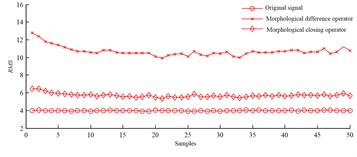a) Root mean square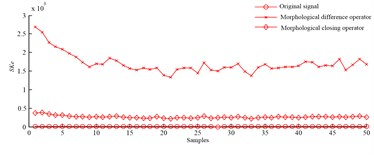b) Skewness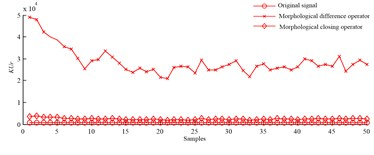c) Kurtosis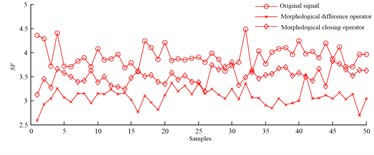d) Shape factor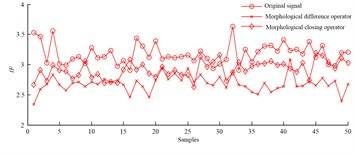e) Impulse factor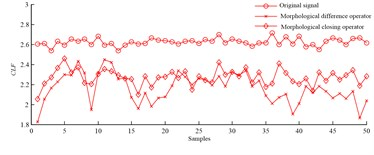f) Clearance factor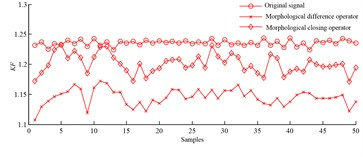g) Kurtosis factor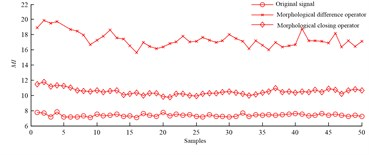h) Morphological index

Slipper fault signals are filtered by morphological difference operator and morphological closing operator respectively, and root mean square, skew, kurtosis and morphological index are taken from filtered signals. It is can be found from Fig. 1 that the parameter values extracted from fault signals based on the two operators don’t overlap with each other, and the parameter values also don’t overlap with those of normal signals. It should be pointed out that parameter values extracted based on morphological difference operator are much bigger than those obtained based on morphological closing operator, and value of morphological index firstly introduced into hydraulic motor fault field is bigger than that of root mean square. The conclusion is that the four parameters are all sensitive to slipper fault, thus root mean square, skew, kurtosis and morphological index can all be applied to diagnose slipper fault, and morphological index is more sensitive than root mean square.

Based on morphological difference operator, shape factor, impulse factor, clearance factor and kurtosis factor are extracted from abnormal signals, and their values don’t overlap with those extracted from normal signals. Conclusions drawn from above demonstrate that these parameters are sensitive to slipper fault, and they can diagnose slipper fault effectively.

Based on method of morphological difference operator, shape factor, impulse factor, and kurtosis factor can be obtained from abnormal signals, and their values overlap with those extracted from normal signals in some degree. Conclusions are summarized from above and show that these parameters are not so much sensitive to slipper fault, and slipper fault can’t be diagnosed effectively.

Clearance factor are extracted from abnormal and normal signals based on morphological difference operator and morphological closing operator, and abnormal and normal values don’t overlap with each other, and the parameter is sensitive to slipper fault. What need to be explained are differences between abnormal values and normal ones extracted based on morphological difference operator are bigger than those extracted based on morphological closing operator. Thus, the fault can be diagnosed by the parameter.

Value differences between normal and abnormal parameter extracted based on morphological difference operator is shorted for A, and value differences between normal and abnormal parameter extracted based on morphological closing operator is shorted for B, and A is bigger than B in some degree. Thus, morphological difference operator is prior to morphological closing operator in time domain.

### 3.2. Extraction of frequency domain parameters

Frequency domain parameters are extracted from abnormal and normal signals based on morphological difference operator and morphological closing operator, and Fig. 1 displays sensitive distribution of 50 samples of each parameter.

Centroid frequency and mean square frequency are extracted from abnormal signals based on both operators, and it can be known from Fig. 2 that their values don’t overlap those extracted from normal signals. The both parameters are sensitive to slipper fault, and the fault can be diagnosed by the two parameters.

Frequency variance is extracted from abnormal signals based on morphological difference operator, and their values don’t overlap with those obtained from normal signals, and therefore the parameter is sensitive to the fault and can diagnose it.

Frequency variance is extracted from abnormal signals based on morphological closing operator, and their values overlap with those got from normal signals in some degree, so the parameter isn’t sensitive to the fault and can’t diagnose it.

Based on morphological closing operator, only frequency variance values extracted from abnormal signals overlap with those extracted from normal signals. And the three parameters extracted based on morphological difference operator all work for the fault diagnosis. Thus, morphological difference operator outperforms morphological closing operator.

Fig. 2Sensitive distribution of frequency domain parameters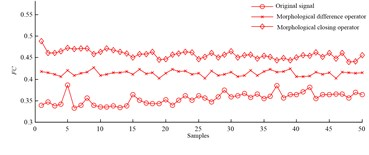a) Centroid frequency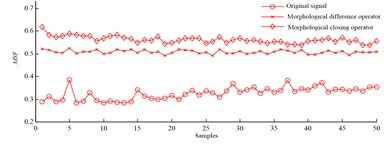b) Mean square frequency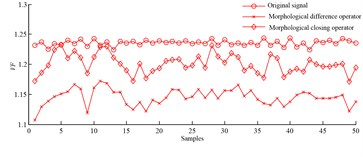c) Frequency variance

## 4. Conclusions

Fusion method based on mathematical morphology and sensitive parameters are put forward, and the method processes self-adaptive filter advantage of mathematical morphology and sensitivity advantage of parameters. Sensitivities of time and frequency parameters to slipper fault are firstly analyzed in fault diagnosis of hydraulic motor, and conclusions of this investigation are summarized as follows.

1) Morphological difference operator is prior to morphological closing operator.

2) Morphological index is firstly introduced into fault diagnosis of hydraulic motor, and the one extracted based on morphological difference operator is sensitive to slipper fault, and fault impulsive power extracted by the operator is much more than that extracted by morphological closing operator.

3) Parameters in time and frequency domains are sensitive to slipper fault, and fault can be diagnosed by them, and fault diagnosis methods based on the time and frequency domain parameters get fully rich.